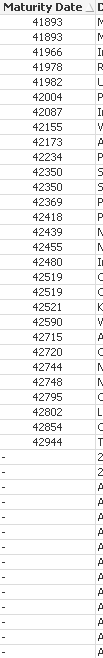# New to QlikView

Discussion board where members can get started with QlikView.

HighlightedNot applicable

## How to show only null values - straight table

I have a straight table and I wan't to filter the table so that only the null values for the field 'Maturity Date' are shown. I wan't to do this as some sort of calculation or expression rather then using the load script or a button. Any ideas?

TIATags (5)
1 Solution

Accepted SolutionsMVP

## Re: How to show only null values - straight table

May be a calculated Dimension:

If(Len(Trim([Maturity Date])) = 0, 'Null', Null())

3 RepliesMVP

## Re: How to show only null values - straight table

May be a calculated Dimension:

If(Len(Trim([Maturity Date])) = 0, 'Null', Null())

Contributor III

## Re: How to show only null values - straight table

Apply set analysis with

{\$<Maturity Date-={*}>} in the expression

Honored Contributor

## Re: How to show only null values - straight table

Hi Ben,

If you have an ID field in your fact table try this as a calculated dimension:

=Aggr(Alt([Maturity Date],'Null'),ID)

If you pick 'Null' in this calculated dimension Qlikview will select the IDs for which there is no Maturity Date.

good luck

Andrew